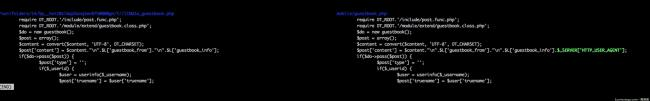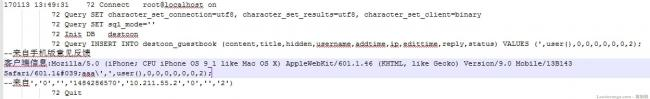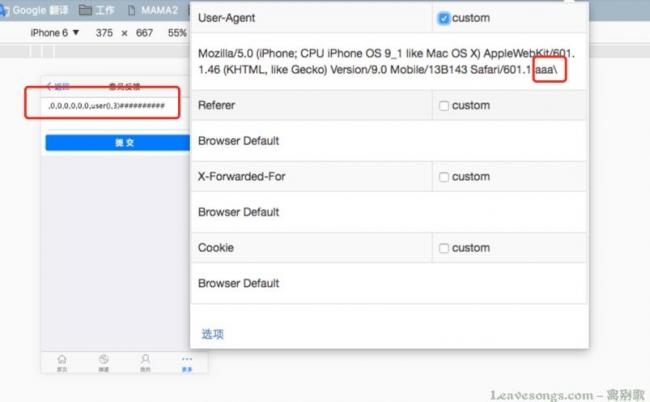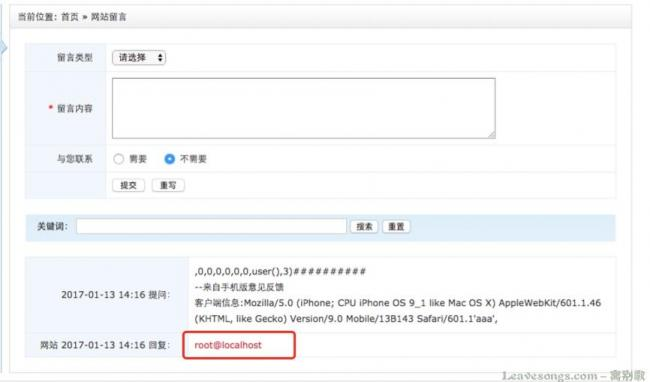## 补丁分析mobile/guestbook.php 中将`\$_SERVER['HTTP_USER_AGENT']`删掉了。分析一下，这里是手机端的留言板，destoon将用户的User-Agent放入了留言内容变量`\$post[content]`中。

```<?php
\$post = \$this->set(\$post);
\$sqlk = \$sqlv = '';
foreach(\$post as \$k=>\$v) {
if(in_array(\$k, \$this->fields)) { \$sqlk .= ','.\$k; \$sqlv .= ",'\$v'"; }
}
\$sqlk = substr(\$sqlk, 1);
\$sqlv = substr(\$sqlv, 1);
\$this->db->query("INSERT INTO {\$this->table} (\$sqlk) VALUES (\$sqlv)");
return \$this->itemid;
}
```

```<?php
function set(\$post) {
global \$DT_TIME, \$_username, \$DT_IP, \$TYPE;
\$post['content'] = strip_tags(\$post['content']);
\$post['title'] = in_array(\$post['type'], \$TYPE) ? '['.\$post['type'].']' : '';
\$post['title'] .= dsubstr(\$post['content'], 30);
\$post['hidden'] = isset(\$post['hidden']) ? 1 : 0;
if(\$this->itemid) {
\$post['status'] = \$post['status'] == 2 ? 2 : 3;
\$post['edittime'] = \$DT_TIME;
} else {
\$post['ip'] =  \$DT_IP;
\$post['edittime'] = 0;
\$post['status'] = 2;
}
\$post = dhtmlspecialchars(\$post);
return array_map("trim", \$post);
}
```

1. content 有如下过程：`strip_tags` -> `htmlspecialchars` -> `trim`
2. title 有如下过程：`in_array(\$post['type'], \$TYPE) ? '['.\$post['type'].']' : ''` -> `substr(\$post['content'], 30)` -> `addslashes` -> `trim`## 漏洞利用1. 怎么绕过长度限制，这个后面说
2. 使用这个方法，就一定要以游客身份留言，否则会有更多没意义的键使长度限制更大

## 长度限制绕过

【代码审计】小密圈中，@雨了个雨 师傅提出，登录用户其实是可以注入出管理员账号密码的。

```<?php
if(\$do->pass(\$post)) {
\$post['type'] = '';
if(\$_userid) {
\$post['truename'] = \$user['truename'];
\$post['telephone'] = \$user['telephone'] ? \$user['telephone'] : \$user['mobile'];
\$post['email'] = \$user['mail'] ? \$user['mail'] : \$user['email'];
\$post['qq'] = \$user['qq'];
\$post['msn'] = \$user['msn'];
\$post['ali'] = \$user['ali'];
\$post['skype'] = \$user['skype'];
}
exit('ok');
}
```

==== 分割线 ====

# 赞赏# 评论Karolyn 回复

What's up friends, good paragraph and nice urging commented here, I am
actually enjoying by these.100 回复phithon 回复

@100：对，我为了测试方便，去掉了。嘿铲批量的大佬们可能需要费点脑经了。test001 回复